# eat: Efficiency Analysis Trees

## A brief introduction to production theory field

This vignette is intended to know the main functions of the eat package. Efficiency Analysis Trees is an algorithm that estimates a production frontier in a data-driven environment by adapting regression trees. In this way, techniques from the field of machine learning are incorporated into solving problems in the field of production theory. From the latter, the following terminology is introduced.

Let us consider $$n$$ Decision Making Units (DMUs) to be evaluated. $$DMU_i$$ consumes $$\textbf{x}_i = (x_{1i}, ...,x_{mi}) \in R^{m}_{+}$$ amount of inputs for the production of $$\textbf{y}_i = (y_{1i}, ...,y_{si}) \in R^{s}_{+}$$ amount of outputs. The relative efficiency of each DMU in the sample is assessed with reference to the so-called production possibility set or technology, which is the set of technically feasible combinations of $$(\textbf{x, y})$$. It is defined in general terms as:

$\begin{equation} \Psi = \{(\textbf{x, y}) \in R^{m+s}_{+}: \textbf{x} \text{ can produce } \textbf{y}\} \end{equation}$

Monotonicity (free disposability) of inputs and outputs is assumed, meaning that if $$(\textbf{x, y}) \in \Psi$$, then $$(\textbf{x', y'}) \in \Psi$$, as soon as $$\textbf{x'} \geq \textbf{x}$$ and $$\textbf{y'} \leq \textbf{y}$$. Often convexity of $$\Psi$$ is also assumed. The efficient frontier of $$\Psi$$ may be defined as $$\partial(\boldsymbol{\Psi}) := \{(\boldsymbol{x,y}) \in \boldsymbol{\Psi}: \boldsymbol{\hat{x}} < \boldsymbol{x}, \boldsymbol{\hat{y}} > \boldsymbol{y} \Rightarrow (\boldsymbol{\hat{x},\hat{y}}) \notin \boldsymbol{\Psi} \}$$. Technical inefficiency is defined as the distance from a point that belongs to $$\Psi$$ to the production frontier $$\partial(\Psi)$$. For a point located inside $$\Psi$$, it is evident that there are many possible paths to the frontier, each associated with a different technical inefficiency measure.

## Summary of eat functions

In this section, an EAT model, a RFEAT model, a FDH model and a DEA model refer to a modeling carried out using Efficiency Analysis Trees technique, Random Forest for Efficiency Analysis Trees technique, Free Disposal Hull method and Data Envelopment Analysis method, respectively. Additionally, a CEAT model refers to a Convexified Efficiency Analysis Trees model. The functions developed in the eat library are always oriented to one of the five previous models (EAT, CEAT, RFEAT, FDH or DEA) and can be divided into seven categories depending on their purpose:

Purpose Function.name Usage
Model EAT It generates a pruned Efficiency Analysis Trees model and returns an EAT object.
Model RFEAT It generates a Random Forest for Efficiency Analysis Trees model and returns a RFEAT object
Summarize print Print method for an EAT or a RFEAT object.
Summarize summary Summary method for an EAT object.
Summarize EAT_size For an EAT object. It returns the number of leaf nodes.
Summarize EAT_frontier_levels For an EAT object. It returns the frontier output levels at the leaf nodes.
Summarize EAT_leaf_stats For an EAT object. It returns a descriptive summary statistics table for each output variable calculated from the leaf nodes observations.
Tune bestEAT For an EAT model. Hyperparameter tuning.
Tune bestRFEAT For an RFEAT model Hyperparameter tuning.
Graph frontier For an EAT object. It plots the estimated frontier in a two-dimensional scenario (1 input and 1 output).
Graph plotEAT For an EAT object. It plots the tree structure.
Graph plotRFEAT For an RFEAT object. It plots a line plot graph with the Out-of-Bag (OOB) error for a forest consisting of k trees.
Calculate efficiency scores efficiencyEAT It calculates the efficiency scores through an EAT (and FDH) model.
Calculate efficiency scores efficiencyCEAT It calculates the efficiency scores through a convexified EAT (and DEA) model.
Calculate efficiency scores efficiencyRFEAT It calculates the efficiency scores through a RFEAT (and FDH) model.
Graph efficiency scores efficiencyDensity Density plot for a data.frame of efficiency scores (EAT, FDH, CEAT, DEA and RFEAT are available).
Graph efficiency scores efficiencyJitter For an EAT object. Jitter plot for a vector of efficiency scores calculated through an EAT /CEAT model.
Predict predict Predict method for an EAT or a RFEAT object.
Rank rankingEAT For an EAT object. It calculates variable importance scores.
Rank rankingRFEAT For an RFEAT object. It calculates variable importance scores.
Simulation Y1.sim It simulates a data set in 1 output scenario. 1, 3, 6, 9, 12 and 15 inputs can be generated.
Simulation X2Y2.sim It simulates a data set in 2 outputs and 2 inputs scenario.

## The PISAindex database

The PISAindex database is included as a data object in the eat library and is employed to exemplify the package functions. On the one hand, the inputs correspond to 13 variables that define the socioeconomic context of a country, by means of a score in the range [1-100], except for the Gross Domestic Product by Purchasing Power Parity, which is measured in thousands of dollars. All of them have been obtained from the Social Progress Index. On the other hand, the performance of each country in the PISA exams is measured by the average score of its schools in the disciplines of Science, Reading and Mathematics which have been collected from PISA 2018 Results.

The following variables are collected for 72 countries that take the PISA exam:

• Country, Continent and a 3-letter code that identifies the country following the ISO 3166 ALPHA-3 as rownames.

• Outputs :

• S_PISA : mean score on the PISA exam in Science.
• R_PISA : mean score on the PISA exam in Reading.
• M_PISA : mean score on the PISA exam in Mathematics.
• Inputs :

• Basic Human Needs field :

• NBMC : Nutrition and Basic Medical Care.
• WS : Water and Sanitation.
• S : Shelter.
• PS : Personal Safety.
• Foundations of Well-being field :

• HW : Health and Wellness.
• EQ : Environmental Quality.
• Opportunity field :

• PR : Personal Rights.
• PFC : Personal Freedom and Choice.
• I : Inclusiveness.
• GDP_PPP : Gross Domestic Product based on Purchasing Power Parity.

The eat package is applied with the following purposes: (1) to create homogeneous groups of countries in terms of their socioeconomic characteristics (Basic Human Needs, Foundations of Well-being, Opportunity and GDP PPP per capita) and subsequently to know what maximum PISA score is expected in one or more specific disciplines for each of these groups; (2) to know which countries exercise best practices and which of them do not obtain a performance according to their socioeconomic level; and (3) to know what variables are more relevant in obtaining efficient levels of output.

# We save the seed for reproducibility of the results
set.seed(120)
library(eat)
data("PISAindex")

## Modeling a scenario with an input and an output. Plotting the frontier

### EAT()

The EAT function is the centerpiece of the eat library. EAT performs a regression tree based on CART methodology under a new approach that guarantees obtaining a frontier as an estimator that fulfills the property of free disposability. This new technique has been baptized as Efficiency Analysis Trees. The development of the functions contained in the eat library has been designed so that even true R novices can use the library easily. The minimum arguments of the function are the data (data) containing the study variables, the indexes of the predictor variables or inputs (x) and the indexes of the predicted variables or outputs (y). Additionally, the numStop, fold, max.depth and max.leaves arguments are included for those more experienced users in the fields of machine learning and tree-based models. Modifying these four allows obtaining different frontiers and therefore selecting the one that best suits the needs of the analysis.

• numStop refers to the minimum number of observations in a node to be divided and is directly related to the size of the tree. The higher the value of numStop, the smaller the size of the tree.

• fold refers to the number of parts in which the dataset is divided to apply the cross-validation technique. Variations in the fold argument are not directly related to the size of the tree.

• max.depth limits the number of nodes between the root node and the furthest leaf node (root not included). When this argument is introduced, the typical process of growth-pruning is not carried out. In this case, the tree is allowed to grow to the required depth.

• max.leaves determines the maximum number of leaf nodes. As in max.depth, the process of growth-pruning is not performed. In this respect, the tree grows until the required number of leaf nodes is reached, and then, the tree is returned.

Note that including the arguments max.depth or max.leaves hyperparameters reduce the computation time by eliminating the pruning procedure. If both are included at the same time, a warning message is displayed and only max.depth is used.

The error of a given node $$t$$ is measured as the prediction error at the node $$t$$ over the total number of observations:

$\begin{equation} R(t) = \frac{n(t)}{N} \cdot MSE(t) = \frac{1}{N} \cdot \sum_{(x_i,y_i)\in t}(y_i - \hat{y}(t))^2 \end{equation}$

The impurity of a tree $$T$$ is measured as the sum of the impurities for each leaf node:

$\begin{equation} R(T) = \sum_{i = 1}^{\widetilde{T}}R(t_i), \end{equation}$

where $$\widetilde{T}$$ is the set of leaf nodes for the tree $$T$$.

The function returns an EAT object.

EAT(data, x, y,
fold = 5,
numStop = 5,
max.depth = NULL,
max.leaves = NULL,
na.rm = TRUE)
• Example 1: M_PISA ~ PFC
single_model <- EAT(data = PISAindex,
x = 15, # input
y = 3) # output

print() returns the tree structure for an EAT object where:

• y : vector of predictions.
• R : error at the node.
• n(t) : number of DMUs at the node.
• input name < / >= s represents the division of the space.
• <*> indicates a leaf node.
print(single_model)
  y: [ 551 ] || R: 11507.5 n(t): 72

|   PFC < 77.2 --> y: [ 478 ] || R: 2324.47 n(t): 34

|   |   PFC < 65.45 --> y: [ 428 ] <*> || R: 390.17 n(t): 16

|   |   PFC >= 65.45 --> y: [ 478 ] <*> || R: 637.08 n(t): 18

|   PFC >= 77.2 --> y: [ 551 ] <*> || R: 2452.83 n(t): 38

<*> is a leaf node

summary() returns the following information of an EAT object:

• Formula : outputs ~ inputs

• Summary for leaf nodes where:

• id : leaf node index.
• n(t) : number of DMUs at the leaf node.
• % : proportion of DMUs at the leaf node.
• As many columns as output names with the corresponding predictions for the leaf nodes.
• R(t) : error at the leaf node.
• Tree where:

• Interior nodes : number of interior nodes.
• Leaf nodes : number of leaf nodes.
• Total nodes : total number of nodes (interior nodes + leaf nodes).
• R(T) : error at the model.
• numStop : numStop hyperparameter value.
• fold : fold hyperparameter value.
• max.depth : max.depth hyperparameter value.
• max.leaves : max.leaves hyperparameter value.
• Primary & surrogate splits where:

• Node A --> {B, C} indicates that the node A is split into the left child node B and the right child node C.
• variable --> {R: , s: } represents the division of the space with its error and threshold.
• Surrogate splits indicates the best possible split for each variable that has not been used to divide the node. This is expressed as variable --> {R: , s: } where R is the error at the node and s the threshold. Results are displayed in descending order by their R value. In the case of a single input, the surrogate splits do not appear.
summary(single_model)

Formula:  S_PISA ~ PFC

# ========================== #
#   Summary for leaf nodes   #
# ========================== #

id n(t)  % S_PISA    R(t)
3   38 53    551 2452.83
4   16 22    428  390.17
5   18 25    478  637.08

# ========================== #
#            Tree            #
# ========================== #

Interior nodes: 2
Leaf nodes: 3
Total nodes: 5

R(T): 3480.08
numStop: 5
fold: 5
max.depth:
max.leaves:

# ========================== #
# Primary & surrogate splits #
# ========================== #

Node 1 --> {2,3} || PFC --> {R: 4777.31, s: 77.2}

Node 2 --> {4,5} || PFC --> {R: 1027.25, s: 65.45}

EAT_size() returns the number of leaf nodes of an EAT model:

EAT_size(single_model)
The number of leaf nodes of the EAT model is: 3
 3

EAT_frontier_levels() returns the frontier levels of the outputs at the leaf nodes:

EAT_frontier_levels(single_model)
The frontier levels of the outputs at the leaf nodes are:  
  S_PISA
1    551
2    428
3    478

EAT_leaf_stats() returns a descriptive summary statistics table for each output variable calculated from the leaf nodes observations of an Efficiency Analysis Trees model. In multioutput scenarios, the measurements are shown for each output. Specifically, it computes:

• Node: node index.
• n(t): number of DMUs.
• %: proportion of DMUs.
• mean: mean.
• var: variance.
• sd: standard deviation.
• min: minimum.
• Q1: first quantile.
• median: median.
• Q3: third quantile.
• max: maximum.
• RMSE: root mean square error.
EAT_leaf_stats(single_model)
  Node n(t)   %   mean     var    sd min     Q1 median     Q3 max   RMSE
1    1   72 100 455.06 2334.59 48.32 336 416.75  466.0 495.25 551 107.27
2    2   34  47 416.88 1223.02 34.97 336 397.25  415.5 435.75 478  70.16
3    3   38  53 489.21  851.95 29.19 419 478.00  494.0 504.50 551  68.17
4    4   16  22 394.62  684.65 26.17 336 381.50  398.0 414.00 428  41.90
5    5   18  25 436.67  889.29 29.82 386 415.25  433.5 468.00 478  50.48

Additionally, EAT_object[["tree"]][[id_node]] or EAT_object$tree[[id_node]] returns a list that allows knowing the characteristics of a given node in greater detail. The elements that define a node are the following: • id : node index. • F : father node index. • SL : left child node index. • SR : right child node index. • index : set of indexes corresponding to the observations in a node. • varInfo : list containing the error of the left node, the error of the right node and the threshold of the best split for each input. • R : error at the node. • xi : index of the variable that produces the split in a node. • s : threshold of the variable xi by which the split takes place. • y : value(s) of the predicted variable(s) in a node. • a : lower bound of the given node. • b : upper bound of the given node. Note that: • The node with index 1 has a value of -1 in F since it has no parent node. • A leaf node has a value of -1 in SL, SR, s and xi since it is not divided. single_model[["tree"]][] $id
 5

$F  2$SL
 -1

$SR  -1$index
 26 27 34 36 38 39 41 42 43 45 47 48 49 58 59 65 66 68

$varInfo$varInfo[]
$varInfo[][]  363.2778$varInfo[][]
 759.8056

$varInfo[][]  64.32$R
 637.0833

$xi  -1$s
 -1

$y$y[]
 478

$a  65.45$b
 77.2

### Categorical variables

The types of variables accepted by the EAT function are the following:

Variable Integer Numeric Factor Ordered.factor
Independent variables (inputs) x x x
Dependent variables (outputs) x x

The Efficiency Analysis Trees methodology does not allow categorical variables. At this time, only ordinal factors can be entered. It is important to note that order = True must be included in the factor construction so as not to produce an error.

• Factor (not ordered)
# Transform Continent to Factor
PISAindex_factor_Continent <- PISAindex
PISAindex_factor_Continent$Continent <- as.factor(PISAindex_factor_Continent$Continent)
error_model <- EAT(data = PISAindex_factor_Continent,
x = c(2, 15),
y = 3) 
Error in preProcess(data = data, x = x, y = y, numStop = numStop, fold = fold, : Continent is not a numeric, integer or ordered vector
• Factor ordered
# Cateogirze GDP_PPP into 4 groups: Low, Medium, High, Very High.
PISAindex_GDP_PPP_cat <- PISAindex

PISAindex_GDP_PPP_cat$GDP_PPP_cat <- cut(PISAindex_GDP_PPP_cat$GDP_PPP,
breaks = c(0, 16.686, 31.419, 47.745, Inf),
include.lowest = T,
labels = c("Low", "Medium", "High", "Very high"))

class(PISAindex_GDP_PPP_cat$GDP_PPP_cat) # "factor" --> error  "factor" # It is necessary to indicate order = TRUE, before applying the EAT function PISAindex_GDP_PPP_cat$GDP_PPP_cat <- factor(PISAindex_GDP_PPP_cat$GDP_PPP_cat, order = TRUE) class(PISAindex_GDP_PPP_cat$GDP_PPP_cat) # "ordered" "factor" --> correct
 "ordered" "factor" 
categorized_model <- EAT(data = PISAindex_GDP_PPP_cat,
x = c(15, 19),
y = 3) 

### frontier()

The frontier function displays the frontier estimated by the EAT function through a plot from ggplot2. The frontier estimated by FDH can also be plotted if FDH = TRUE. Observed DMUs can be showed by a scatterplot if observed.data = TRUE and its color, shape and size can be modified with observed.color, pch and size respectively. Finally, rownames can be included with rwn = TRUE.

frontier(object,
FDH = FALSE,
observed.data = FALSE,
observed.color = "black",
pch = 19,
size = 1,
rwn = FALSE,
max.overlaps = 10)

To continue, the frontier of the previous model is displayed. It can be seen how the frontier obtained by the EAT function generalizes the results of the frontier obtained through FDH, thus avoiding overfitting. The boundary estimated through Efficiency Analysis Trees generates 3 steps corresponding to the 3 leaf nodes (nodes 3, 4 and 5) obtained with the EAT function. For each of these steps, a frontier level in terms of the output is given with respect to the amount of input used (in this case level of PFC). In addition, we can appreciate 6 DMUs on the frontier: ALB (Albania), MDA (Moldova), SRB (Serbia), RUS (Russia), HUN (Hungary) and SGP (Singapore). Note that the first vertical plane of the frontier does not appear, but if it did, ALB would be on it. These DMUs are efficient and the rest of the DMUs below their specific step should increase the amount of output obtained or reduce the amount of input utilized until reaching the boundary to be efficient.

frontier <- frontier(object = single_model,
FDH = TRUE,
observed.data = TRUE,
rwn = TRUE)

plot(frontier)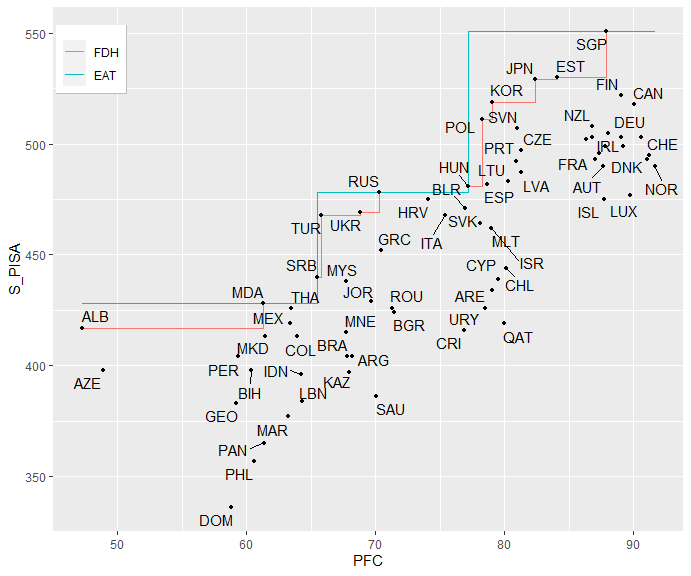• Is the number of steps on the frontier always the same as the number of leaf nodes?

The answer is no. Note that there may be situations where the estimation of two or more nodes is identical. This is necessary to ensure the estimation of an increasing monotonic frontier. In this case, the number of leaf nodes is 5, however the predictions for nodes 4 and 5 are the same and therefore the border only has 4 steps.

single_model_md <- EAT(data = PISAindex,
x = 15,
y = 3,
max.leaves = 5) 
EAT_size(single_model_md)
The number of leaf nodes of the EAT model is: 5
 5
single_model_md[["model"]][["y"]]
     [,1]
[1,]  551
[2,]  478
[3,]  428
[4,]  417
[5,]  417
frontier_md <- frontier(object = single_model_md,
observed.data = TRUE)

plot(frontier_md)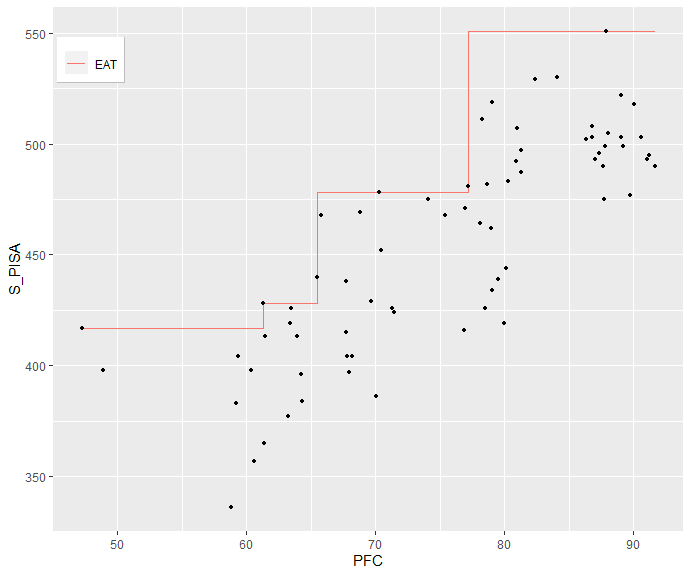## Modeling a multioutput scenario. Feature selection.

• Example 2: S_PISA + R_PISA + M_PISA ~ NBMC + WS + S + PS + ABK + AIC + HW + EO + PR + PFC + I + AAE + GDP_PPP
multioutput_model <- EAT(data = PISAindex,
x = 6:18,
y = 3:5
) 

### rankingEAT()

The second example presents a multiple output scenario where 13 inputs are used to model the 3 available outputs. In these situations, a selection of the most contributing variables may be recommended in order to reduce overfitting, improve precision and reduce future training times. rankingEAT() allows a selection of variables by calculating a score of importance through the Efficiency Analysis Trees technique. The user can specify the number of decimal units (digits), include a barplot with the scores of importance (barplot) and display a horizontal line in the graph to facilitate the cut-off point between important and irrelevant variables (threshold).

rankingEAT(object,
barplot = TRUE,
threshold = 70,
digits = 2)

The importance score represents how influential each variable is in the model. In this case, the cut-off point is set at 70 and therefore important variables are considered: AAE (Access to Advanced Education), WS (Water and Sanitation), NBMC (Nutrition and Basic Medical Care), HW (Health and Wellness) and S (Shelter).

rankingEAT(object = multioutput_model,
barplot = TRUE,
threshold = 70,
digits = 2)
$scores Importance AAE 100.00 WS 97.65 NBMC 82.55 HW 82.49 S 82.24 ABK 67.16 GDP_PPP 64.54 AIC 64.08 EQ 56.29 PR 56.22 I 56.22 PS 45.38 PFC 29.35$barplot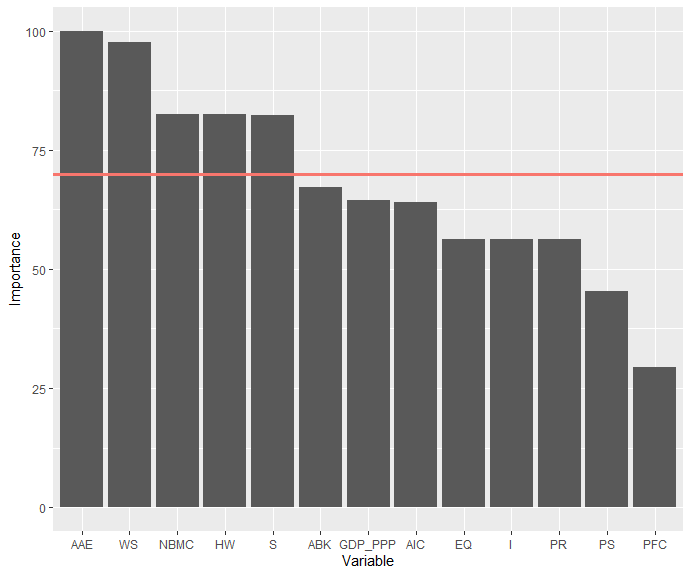## Graphical representation by a tree structure

### plotEAT()

frontier() allows us to clearly see the regions of the input space originated with EAT(); however, this is impossible with more than two variables (one input and one output). For multiple input and / or output scenarios, the typical tree-structure showing the relationships between the predicted and predictive variables, is given.

In each node, we can obtain the following information:

• id: node index.
• R: error at the node.
• n(t): number of DMUs at the node.
• input name by which the split take place.
• y : vector of predictions.

Furthermore, the nodes are colored according to the variable by which the division is performed or they are black, in the case of being a leaf node.

plotEAT(object)

Below are the 3 ways to control the size of a tree model: numStop, max.depth and max.leaves.

• Example 3: S_PISA + R_PISA + M_PISA ~ NBMC + WS + S + HW + AAE

Size control by numStop

reduced_model1 <- EAT(data = PISAindex,
x = c(6, 7, 8, 12, 17),
y = 3:5,
numStop = 9)
plotEAT(object = reduced_model1)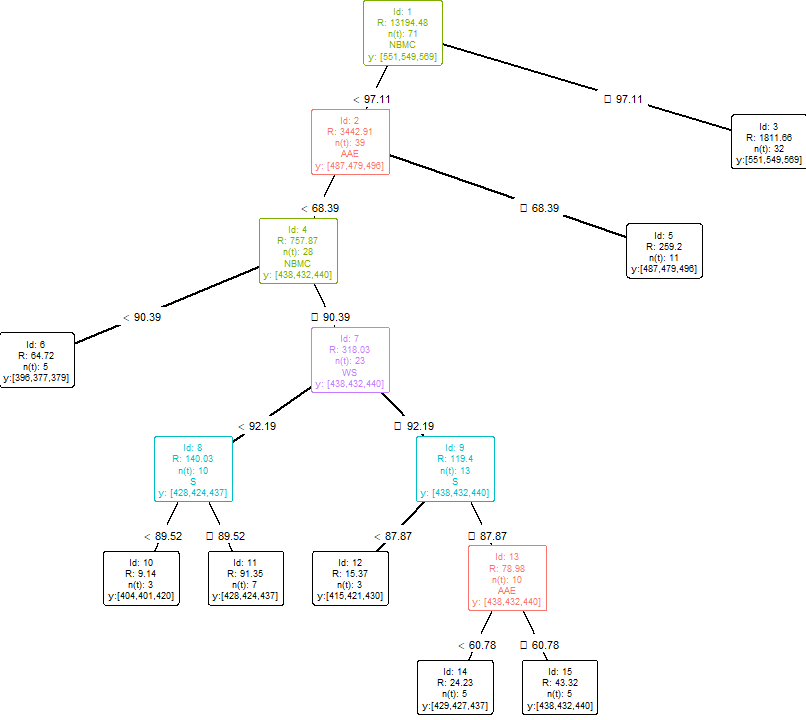# Leaf nodes: 8
# Depth: 6

Size control by max.depth

reduced_model2 <- EAT(data = PISAindex,
x = c(6, 7, 8, 12, 17),
y = 3:5,
numStop = 9,
max.depth = 5)
plotEAT(object = reduced_model2)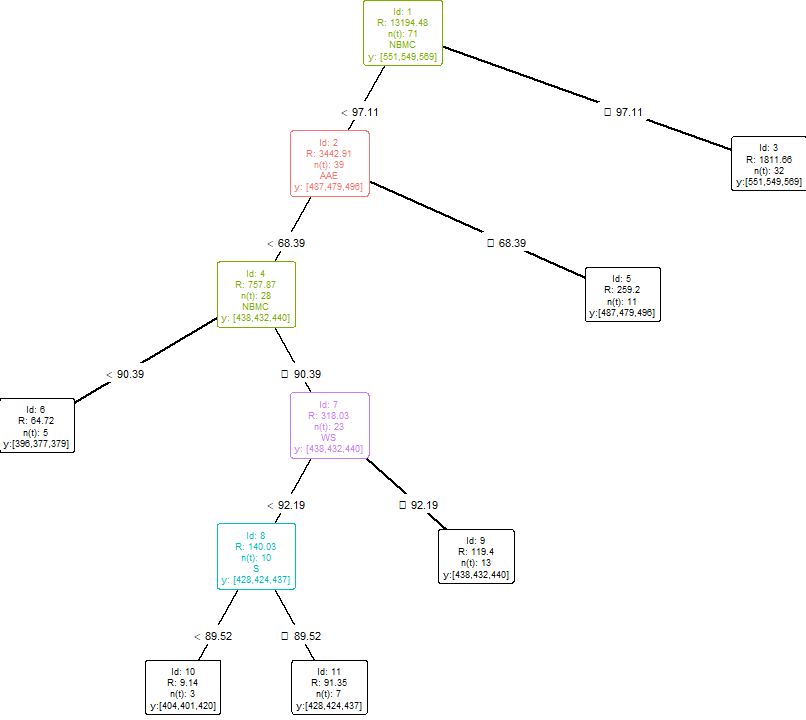# Leaf nodes: 6
# Depth: 5

Size control by max.leaves

reduced_model3 <- EAT(data = PISAindex,
x = c(6, 7, 8, 12, 17),
y = 3:5,
numStop = 9,
max.leaves = 4)
plotEAT(object = reduced_model3)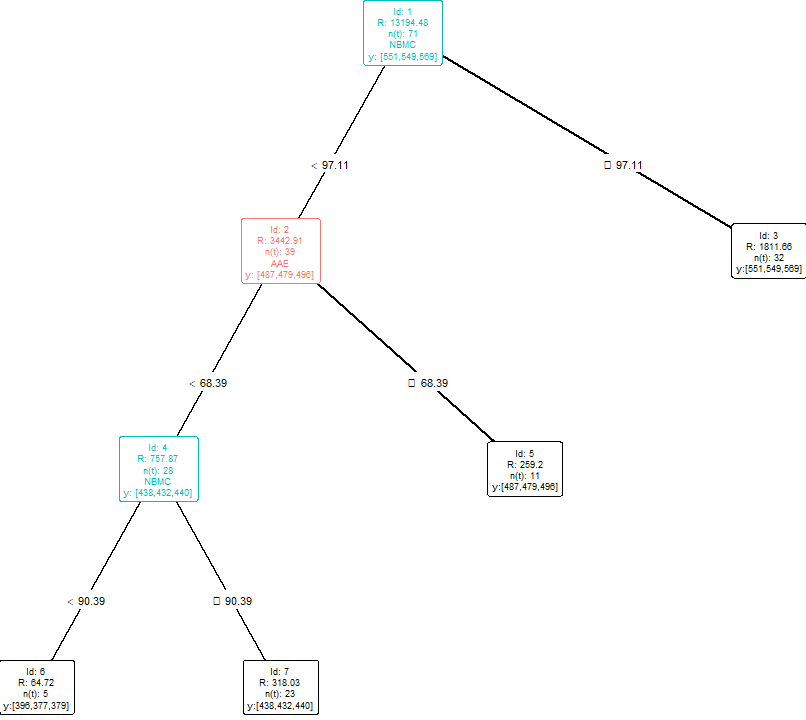# Leaf nodes: 4
# Depth: 3

## The EAT hyperparameter tuning

In this section, the PISAindex database is divided into a training subset with 70% of the DMUs and a test subset with the remaining 30%. Next, the bestEAT function is applied to find the value of the hyperparameters that minimize the error calculated on the test sample from an Efficiency Analysis Trees fitted with the training sample.

n <- nrow(PISAindex) # Observations in the dataset
selected <- sample(1:n, n * 0.7) # Training indexes
training <- PISAindex[selected, ] # Training set
test <- PISAindex[- selected, ] # Test set

### bestEAT()

The bestEAT function requires a training set (training) on which to model an Efficiency Analysis Trees model (with cross-validation) and a test set (test) on which to calculate the error. The number of trees built is given by the number of different combinations that can be given by the numStop, fold, max.depth and max.leaves arguments. Note that it is not possible to enter NULL and a certain value in max.depth or max.leaves arguments at the same time. bestEAT() returns a data frame with the following columns:

• numStop : numStop hyperparameter value.
• fold : fold hyperparameter value.
• max.depth : max.depth hyperparameter value if it does not set to NULL.
• max.leaves : max.leaves hyperparameter value if it does not set to NULL.
• RMSE: root mean square error calculated on the test sample with a tree built with the training sample and the set of hyperparameters.
• leaves: number of leaf nodes at the tree.
bestEAT(training, test,
x, y,
numStop = 5,
fold = 5,
max.depth = NULL,
max.leaves = NULL,
na.rm = TRUE)

For example, if the arguments numStop = {3, 5, 7} and fold = {5, 7} are entered, 6 models of Efficiency Analysis Trees are constructed with {numStop = 3, fold = 5}, {numStop = 3, fold = 7}, {numStop = 5, fold = 5}, {numStop = 5, fold = 7}, {numStop = 7, fold = 5} and {numStop = 7, fold = 7}.

Hyperparameter tuning for:

S_PISA + R_PISA + M_PISA ~ NBMC + WS + S + HW + AAE.

numStop = {3, 5, 7} and fold = {5, 7}

bestEAT(training = training,
test = test,
x = c(6, 7, 8, 12, 17),
y = 3:5,
numStop = c(3, 5, 7),
fold = c(5, 7))
  numStop fold  RMSE leaves
1       3    7 56.82     24
2       3    5 58.81     13
3       7    5 59.14     10
4       7    7 59.14     10
5       5    7 60.13     12
6       5    5 60.24     11

The best Efficiency Analysis Trees model is given by the hyperparameters {numStop = 3, fold = 7} with RMSE = 56.82 and 24 leaf nodes. However, this model is too complex. Therefore, we select the model with parameters {numStop = 7, fold = 5} with RMSE = 59.14 but with only 10 leaf nodes. Now we check the results of this model.

bestEAT_model <- EAT(data = PISAindex,
x = c(6, 7, 8, 12, 17),
y = 3:5,
numStop = 7,
fold = 5)
summary(bestEAT_model)

Formula:  S_PISA + R_PISA + M_PISA ~ NBMC + WS + S + HW + AAE

# ========================== #
#   Summary for leaf nodes   #
# ========================== #

id n(t)  % S_PISA R(t) M_PISA       R
3   32 45    551  549    569 1811.66
6    5  7    396  377    379   64.72
10    3  4    404  401    420    9.14
11    7 10    428  424    437   91.35
12    3  4    415  421    430   15.37
14    5  7    429  427    437   24.23
15    5  7    438  432    440   43.32
16    3  4    469  466    454   65.48
17    8 11    487  479    496  112.99

# ========================== #
#            Tree            #
# ========================== #

Interior nodes: 8
Leaf nodes: 9
Total nodes: 17

R(T): 2238.26
numStop: 7
fold: 5
max.depth:
max.leaves:

# ========================== #
# Primary & surrogate splits #
# ========================== #

Node 1 --> {2,3} || NBMC --> {R: 5254.57, s: 97.11}
Surrogate splits
AAE --> {R: 5614.76, s: 70.12}
WS --> {R: 6246.35, s: 96.47}
S --> {R: 6360.39, s: 94.88}
HW --> {R: 6647.59, s: 73.95}

Node 2 --> {4,5} || AAE --> {R: 1017.07, s: 68.39}
Surrogate splits
NBMC --> {R: 1890.36, s: 93.68}
S --> {R: 2098.31, s: 90.53}
WS --> {R: 2341.68, s: 86.44}
HW --> {R: 2783.94, s: 57.95}

Node 4 --> {6,7} || NBMC --> {R: 382.76, s: 90.39}
Surrogate splits
HW --> {R: 514.17, s: 59.25}
AAE --> {R: 560.01, s: 50.5}
WS --> {R: 581.11, s: 90.02}
S --> {R: 639.65, s: 83.49}

Node 5 --> {16,17} || NBMC --> {R: 178.47, s: 95.37}
Surrogate splits
S --> {R: 216.28, s: 93.2}
WS --> {R: 237.44, s: 91.21}
AAE --> {R: 240.5, s: 72.76}
HW --> {R: 244.73, s: 68}

Node 7 --> {8,9} || WS --> {R: 259.43, s: 92.19}
Surrogate splits
S --> {R: 275.31, s: 87.87}
AAE --> {R: 278.71, s: 60.78}
NBMC --> {R: 282.77, s: 94.49}
HW --> {R: 299.96, s: 59.25}

Node 8 --> {10,11} || S --> {R: 100.48, s: 89.52}
Surrogate splits
NBMC --> {R: 115.66, s: 94.6}
WS --> {R: 120.97, s: 90.02}
HW --> {R: 121.5, s: 72.35}
AAE --> {R: 128.7, s: 54.17}

Node 9 --> {12,13} || S --> {R: 94.35, s: 87.87}
Surrogate splits
AAE --> {R: 98.11, s: 60.78}
HW --> {R: 114.28, s: 67.69}
NBMC --> {R: 114.97, s: 94.49}
WS --> {R: 115.91, s: 94.63}

Node 13 --> {14,15} || AAE --> {R: 67.55, s: 60.78}
Surrogate splits
S --> {R: 71.15, s: 92.12}
NBMC --> {R: 74.55, s: 94.49}
WS --> {R: 75.49, s: 94.63}
HW --> {R: 76.2, s: 67.69} 

## Efficiency scores. Graphical representation.

### Efficiency Analysis Trees model: efficiencyEAT()

The efficiency scores are numerical values that indicate the degree of efficiency of a set of DMUs. A dataset (data) and the corresponding indexes of input(s) (x) and output(s) (y) must be entered. It is recommended that the dataset with the DMUs whose efficiency is to be calculated coincide with those used to estimate the frontier. However, it is also possible to calculate the efficiency scores for a new dataset. The efficiency scores are calculated using the mathematical programming model included in the argument score_model. The following models are available:

• BCC.OUT: Banker Charnes and Cooper output-oriented radial model. Efficiency level at 1.
• BCC.INP: Banker Charnes and Cooper input-oriented radial model. Efficiency level at 1.
• DDF: Directional Distance Function. Efficiency level at 0.
• RSL.OUT: output-oriented Russell Model. Efficiency level at 1.
• RSL.INP: input-oriented Russell Model. Efficiency level at 1.
• WAM.MIP: Weighted Additive Model with Measure of Inefficiency Proportion. Efficiency level at 0.
• WAM.RAM: Weighted Additive Model with Range Adjusted Measure of Inefficiency. Efficiency level at 0.

If FDH = TRUE, scores are also calculated through a FDH model. Finally, a summary descriptive table of the efficiency scores can be displayed with the argument print.table = TRUE.

For this section, the previously created single_model is used:

efficiencyEAT(data, x, y,
object,
score_model,
digits = 3,
FDH = TRUE,
print.table = FALSE,
na.rm = TRUE)
# single_model <- EAT(data = PISAindex, x = 15, y = 3)

scores_EAT <- efficiencyEAT(data = PISAindex,
x = 15,
y = 3,
object = single_model,
scores_model = "BCC.OUT",
digits = 3,
FDH = TRUE,
print.table = TRUE,
na.rm = TRUE)
 Model  Mean Std. Dev. Min    Q1 Median    Q3   Max
EAT 1.114     0.074   1 1.061  1.110 1.110 1.315
FDH 1.081     0.065   1 1.030  1.069 1.069 1.241
scores_EAT
    EAT_BCC_OUT FDH_BCC_OUT
SGP       1.000       1.000
JPN       1.042       1.000
KOR       1.062       1.000
EST       1.040       1.000
NLD       1.095       1.095
POL       1.078       1.000
CHE       1.113       1.113
CAN       1.064       1.064
DNK       1.118       1.118
SVN       1.087       1.024
BEL       1.104       1.062
FIN       1.056       1.056
SWE       1.104       1.104
GBR       1.091       1.091
NOR       1.124       1.124
DEU       1.095       1.095
IRL       1.111       1.069
AUT       1.124       1.082
CZE       1.109       1.044
LVA       1.131       1.066
FRA       1.118       1.075
ISL       1.160       1.116
NZL       1.085       1.043
PRT       1.120       1.055
AUS       1.095       1.054
RUS       1.000       1.000
ITA       1.021       1.021
SVK       1.187       1.037
LUX       1.155       1.155
HUN       1.146       1.000
LTU       1.143       1.060
ESP       1.141       1.075
USA       1.098       1.056
BLR       1.015       1.015
MLT       1.193       1.106
HRV       1.006       1.006
ISR       1.193       1.106
TUR       1.021       1.000
UKR       1.019       1.000
CYP       1.255       1.182
GRC       1.058       1.058
SRB       1.086       1.000
MYS       1.091       1.068
ALB       1.026       1.000
BGR       1.127       1.127
ARE       1.270       1.177
MNE       1.152       1.128
ROU       1.122       1.122
KAZ       1.204       1.179
MDA       1.000       1.000
AZE       1.075       1.048
THA       1.005       1.005
URY       1.293       1.200
CHL       1.241       1.169
QAT       1.315       1.239
MEX       1.021       1.021
BIH       1.075       1.048
CRI       1.149       1.149
JOR       1.114       1.093
PER       1.059       1.032
GEO       1.117       1.089
MKD       1.036       1.036
LBN       1.115       1.115
COL       1.036       1.036
BRA       1.183       1.158
ARG       1.183       1.158
IDN       1.081       1.081
SAU       1.238       1.215
MAR       1.135       1.135
PAN       1.173       1.173
PHL       1.199       1.168
DOM       1.274       1.241
scores_EAT2 <- efficiencyEAT(data = PISAindex,
x = 15,
y = 3,
object = single_model,
scores_model = "BCC.INP",
digits = 3,
FDH = TRUE,
print.table = FALSE,
na.rm = TRUE)

scores_EAT2
    EAT_BCC_INP FDH_BCC_INP
SGP       0.878       1.000
JPN       0.937       1.000
KOR       0.976       1.000
EST       0.918       1.000
NLD       0.867       0.879
POL       0.987       1.000
CHE       0.846       0.858
CAN       0.857       0.878
DNK       0.848       0.859
SVN       0.953       0.966
BEL       0.879       0.891
FIN       0.867       0.925
SWE       0.865       0.877
GBR       0.877       0.889
NOR       0.842       0.854
DEU       0.852       0.863
IRL       0.884       0.896
AUT       0.881       0.893
CZE       0.950       0.963
LVA       0.950       0.963
FRA       0.887       0.899
ISL       0.746       0.801
NZL       0.890       0.902
PRT       0.954       0.967
AUS       0.889       0.901
RUS       0.931       1.000
ITA       0.868       0.873
SVK       0.838       0.843
LUX       0.729       0.783
HUN       1.000       1.000
LTU       0.981       0.995
ESP       0.961       0.974
USA       0.894       0.906
BLR       0.850       0.913
MLT       0.829       0.833
HRV       0.884       0.949
ISR       0.829       0.833
TUR       0.994       1.000
UKR       0.951       1.000
CYP       0.823       0.823
GRC       0.929       0.935
SRB       1.000       1.000
MYS       0.967       0.967
ALB       1.000       1.000
BGR       0.661       0.858
ARE       0.828       0.828
MNE       0.698       0.698
ROU       0.662       0.859
KAZ       0.696       0.696
MDA       0.771       1.000
AZE       0.967       0.967
THA       0.744       0.966
URY       0.602       0.781
CHL       0.817       0.822
QAT       0.591       0.767
MEX       0.746       0.967
BIH       0.782       0.782
CRI       0.615       0.615
JOR       0.940       0.940
PER       0.795       0.795
GEO       0.798       0.798
MKD       0.768       0.768
LBN       0.735       0.735
COL       0.739       0.739
BRA       0.697       0.697
ARG       0.693       0.693
IDN       0.735       0.735
SAU       0.674       0.674
MAR       0.748       0.748
PAN       0.770       0.770
PHL       0.780       0.780
DOM       0.804       0.804

### Convexified Efficiency Analysis Trees model: efficiencyCEAT()

efficiencyCEAT() returns the efficiency scores for the convexified frontier obtained through an Efficiency Analysis Trees model. In this case, if DEA = TRUE, scores are also calculated through a DEA model.

efficiencyCEAT(data, x, y,
object,
score_model,
digits = 3,
DEA = TRUE,
print.table = FALSE,
na.rm = TRUE)
scores_CEAT <- efficiencyCEAT(data = PISAindex,
x = 15,
y = 3,
object = single_model,
scores_model = "BCC.INP",
digits = 3,
DEA = TRUE,
print.table = TRUE,
na.rm = TRUE)
 Model  Mean Std. Dev.   Min    Q1 Median    Q3 Max
CEAT 0.749     0.077 0.591 0.698  0.749 0.749   1
DEA 0.805     0.094 0.599 0.739  0.801 0.801   1
scores_CEAT
    CEAT_BCC_INP DEA_BCC_INP
SGP        0.878       1.000
JPN        0.872       0.986
KOR        0.878       0.989
EST        0.857       0.969
NLD        0.736       0.824
POL        0.862       0.968
CHE        0.697       0.777
CAN        0.768       0.865
DNK        0.693       0.772
SVN        0.821       0.920
BEL        0.735       0.821
FIN        0.787       0.888
SWE        0.724       0.809
GBR        0.750       0.840
NOR        0.680       0.757
DEU        0.723       0.809
IRL        0.731       0.816
AUT        0.712       0.792
CZE        0.788       0.880
LVA        0.758       0.843
FRA        0.725       0.808
ISL        0.669       0.739
NZL        0.769       0.862
PRT        0.776       0.865
AUS        0.754       0.845
RUS        0.846       0.936
ITA        0.756       0.832
SVK        0.717       0.787
LUX        0.660       0.729
HUN        0.779       0.864
LTU        0.768       0.851
ESP        0.755       0.838
USA        0.756       0.846
BLR        0.750       0.826
MLT        0.703       0.771
HRV        0.792       0.875
ISR        0.703       0.771
TUR        0.866       0.953
UKR        0.831       0.916
CYP        0.628       0.678
GRC        0.754       0.822
SRB        0.767       0.829
MYS        0.734       0.792
ALB        1.000       1.000
BGR        0.661       0.691
ARE        0.616       0.663
MNE        0.698       0.698
ROU        0.662       0.701
KAZ        0.696       0.696
MDA        0.771       0.825
AZE        0.967       0.967
THA        0.744       0.787
URY        0.602       0.637
CHL        0.638       0.692
QAT        0.591       0.599
MEX        0.746       0.755
BIH        0.782       0.782
CRI        0.615       0.615
JOR        0.682       0.731
PER        0.795       0.795
GEO        0.798       0.798
MKD        0.768       0.768
LBN        0.735       0.735
COL        0.739       0.739
BRA        0.697       0.697
ARG        0.693       0.693
IDN        0.735       0.735
SAU        0.674       0.674
MAR        0.748       0.748
PAN        0.770       0.770
PHL        0.780       0.780
DOM        0.804       0.804

### efficiencyJitter()

efficiencyJitter returns a jitter plot from ggplot2. This graphic shows how DMUs are grouped into leaf nodes in a model built using the EAT function. Each leaf node groups DMUs with the same level of resources. The dot and the black line represent, respectively, the mean value and the standard deviation of the scores of its node. Additionally, efficient DMU labels are always displayed based on the model entered in the score_model argument. Finally, the user can specify an upper bound (upb) and a lower bound (lwb) in order to show, in addition, the labels whose efficiency score lies between them. Scores from a convex Efficiency Analysis Tree (CEAT) model can also be used.

efficiencyJitter(object, df_scores,
scores_model,
lwb = NULL, upb = NULL)
efficiencyJitter(object = single_model,
df_scores = scores_EAT$EAT_BCC_OUT, scores_model = "BCC.OUT", lwb = 1.2)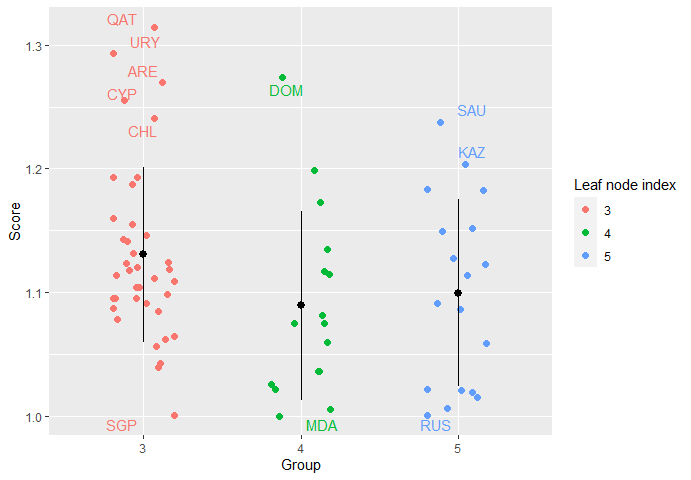efficiencyJitter(object = single_model, df_scores = scores_EAT2$EAT_BCC_INP,
scores_model = "BCC.INP",
upb = 0.65)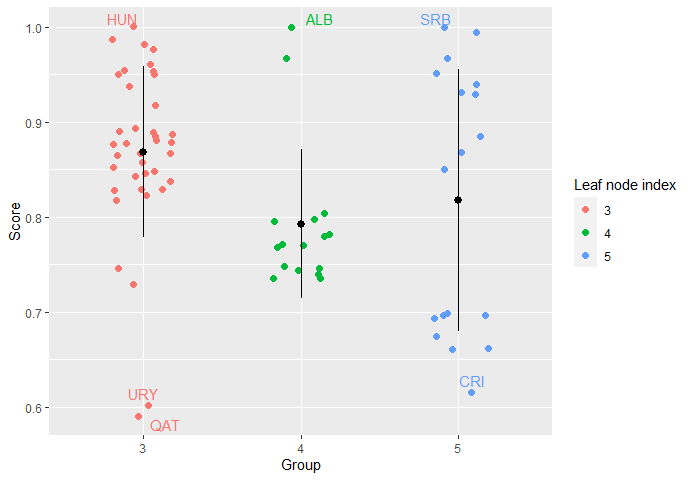Graphically, for a single input and output scenario it is observed that if the BCC models are used to obtain the efficiency scores:

• Under output orientation, those DMUs that are arranged in the horizontal plane of the frontier are efficient.

• Under input orientation those DMUs that are arranged in the vertical plane of the frontier are efficient.

• If a DMU is located in a corner of the frontier, it is efficient under both orientations.

# frontier <- frontier(object = single_model, FDH = TRUE,
# observed.data = TRUE, rwn = TRUE)

plot(frontier)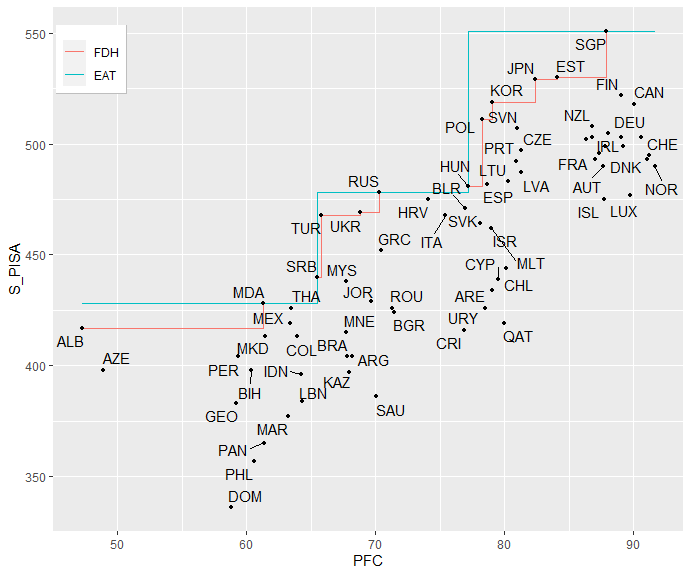### efficiencyDensity()

efficiencyDensity() returns a density plot from ggplot2. In this way, the similarity between the scores obtained by the different available methodologies can be verified.

efficiencyDensity(df_scores,
model = c("EAT", "FDH"))

In this case, we compare EAT vs FDH and CEAT vs DEA:

efficiencyDensity(df_scores = scores_EAT,
model = c("EAT", "FDH"))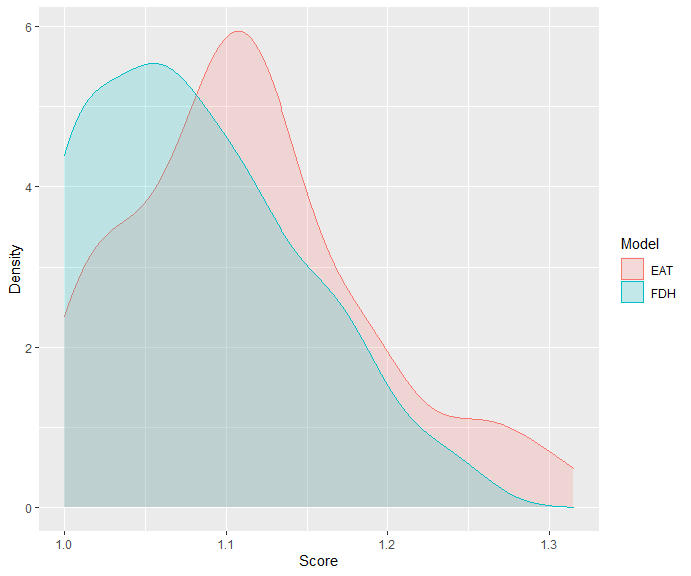efficiencyDensity(df_scores = scores_CEAT,
model = c("CEAT", "DEA"))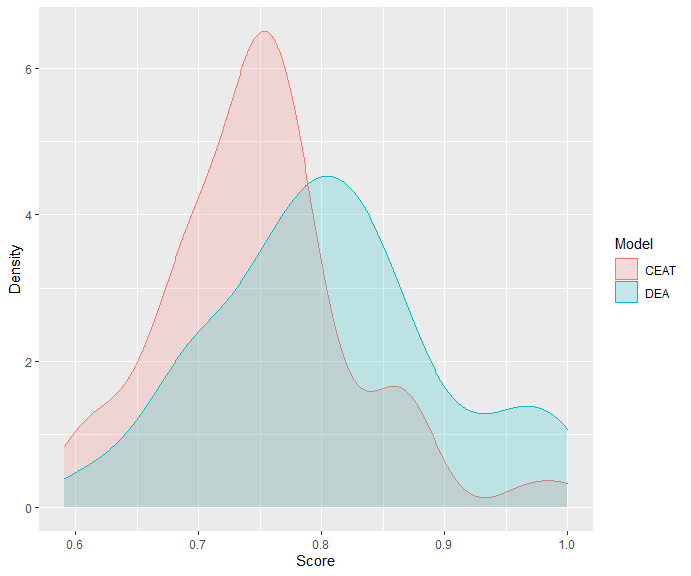The curse of dimensionality

When the ratio of the sample size and the number of variables (inputs and outputs) is low, the standard methods of efficiency analysis (specially FDH) tend to evaluate a large number of DMUs as technically efficient. This problem is known as the curse of dimensionality. To show it, the efficiency scores of the multioutput_model (section 2) with 16 variables and 72 DMUs are calculated:

# multioutput_model <- EAT(data = PISAindex, x = 6:18, y = 3:5)

cursed_scores <- efficiencyEAT(data = PISAindex,
x = 6:18,
y = 3:5,
object = multioutput_model,
scores_model = "BCC.OUT",
digits = 3,
print.table = TRUE,
FDH = TRUE)
 Model  Mean Std. Dev. Min    Q1 Median    Q3   Max
EAT 1.060     0.057   1 1.004  1.047 1.047 1.201
FDH 1.002     0.008   1 1.000  1.000 1.000 1.042
efficiencyDensity(df_scores = cursed_scores, model = c("EAT", "FDH"))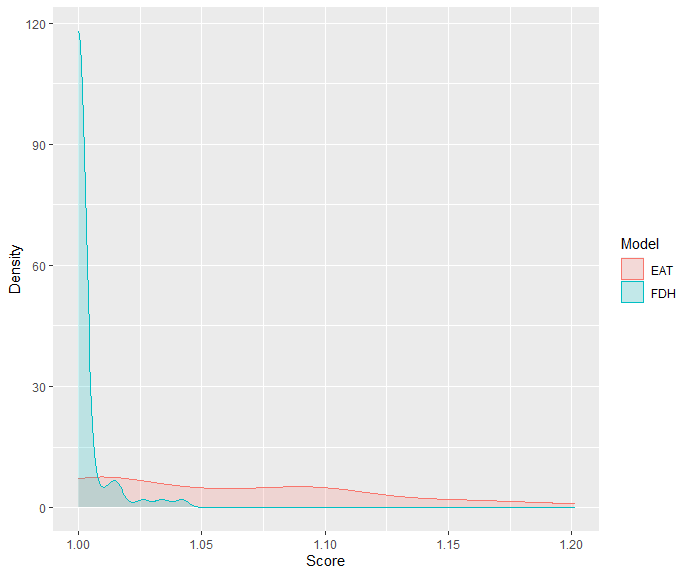## Random Forest

### RFEAT()

Random Forest + Efficiency Analysis Trees (RFEAT) has also been developed with the aim of providing a greater stability to the results obtained by the EAT function. The RFEAT function requires the data containing the variables for the analysis, x and y corresponding to the inputs and outputs indexes respectively, the minimum number of observations in a node for a split to be attempted (numStop) and na.rm to ignore observations with NA cells. All these arguments are used for the construction of the m individual Efficiency Analysis Trees that make up the random forest. Finally, the argument s_mtry indicates the number of inputs that can be randomly selected in each split. It can be set as any integer although there are also certain predefined values. Being, $$n_{x}$$ the number of inputs, $$n_{y}$$ the number of outputs and $$n(t)$$ the number of observations in a node, the available options in s_mtry are:

• BRM = $$\frac{n_{x}}{3}$$
• DEA1 = $$\frac{n(t)}{2} - n_{y}$$
• DEA2 = $$\frac{n(t)}{3} - n_{y}$$
• DEA3 = $$n(t) - 2 \cdot n_{y}$$
• DEA4 = $$min(\frac{n(t)}{n_{y}}, \frac{n(t)}{3} - n_{y})$$

The function returns a RFEAT object.

RFEAT(data, x, y,
numStop = 5, m = 50,
s_mtry = "BRM",
na.rm = TRUE)
forest <- RFEAT(data = PISAindex,
x = 6:18,
y = 3:5,
numStop = 5,
m = 30,
s_mtry = "BRM",
na.rm = TRUE)
print(forest)

Formula:  S_PISA + R_PISA + M_PISA ~ NBMC + WS + S + PS + ABK + AIC + HW + EQ + PR + PFC + I + AAE + GDP_PPP

# ========================== #
#           Forest           #
# ========================== #

Error: 2415.03
numStop: 5
No. of trees (m): 30
No. of inputs tried (s_mtry): BRM

## plotRFEAT()

plotRFEAT() returns the Out-Of-Bag error for a forest consisting of k trees. Note that the OOB error of early forests suffers from great variability.

plotRFEAT(forest)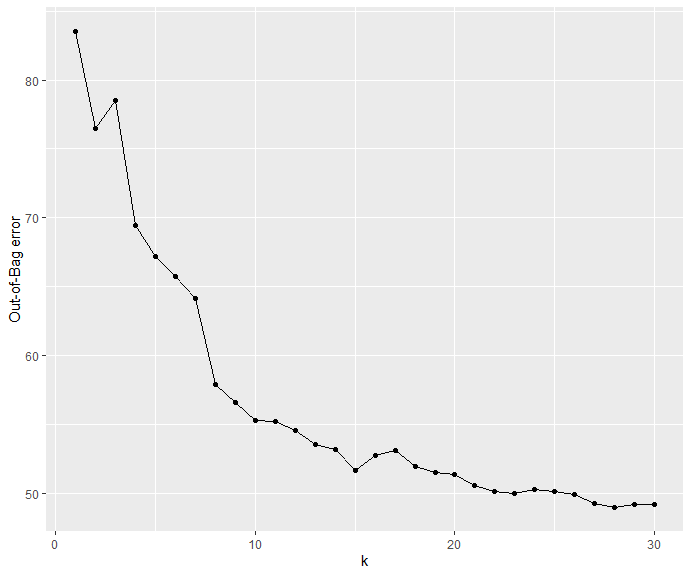## rankingRFEAT()

As in rankingEAT(), the rankingRFEAT function computes an importance score of variables using a RFEAT object:

rankingRFEAT(object,
barplot = TRUE,
digits = 2)

For example (this function is usually computationally exhaustive, thus a previous database reduction is carried out):

forestReduced <- RFEAT(data = PISAindex,
x = c(6, 7, 8, 12, 17),
y = 3:5,
numStop = 5,
m = 30,
s_mtry = "BRM",
na.rm = TRUE)
rankingRFEAT(object = forestReduced,
barplot = TRUE,
digits = 2)
$scores Importance NBMC 7.25 S 3.47 AAE 2.95 HW 2.83 WS -5.58$barplot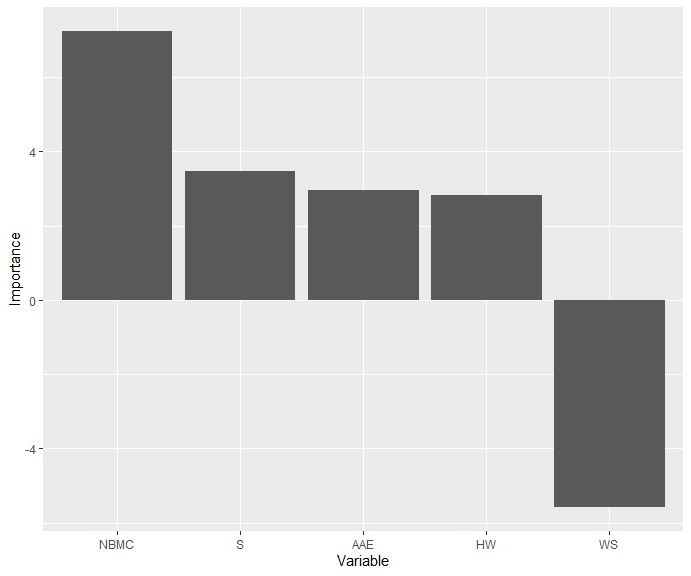• A positive importance score means that including the input in the model improves the performance.

• A negative importance score means that removing the input from the model improves the performance.

## bestRFEAT()

As in bestEAT(), the bestRFEAT function is applied to find the optimal hyperparameters that minimize the root mean square error (RMSE) calculated on the test sample. In this case, the available hyperparameters are numStop, m and s_mtry.

bestRFEAT(training, test,
x, y,
numStop = 5,
m = 50,
s_mtry = c("5", "BRM"),
na.rm = TRUE)

In our example:

# n <- nrow(PISAindex)
# selected <- sample(1:n, n * 0.7)
# training <- PISAindex[selected, ]
# test <- PISAindex[- selected, ]

bestRFEAT(training = training,
test = test,
x = c(6, 7, 8, 12, 17),
y = 3:5,
numStop = c(5, 10), # set of possible numStop
m = c(20, 30), # set of possible m
s_mtry = c("1", "BRM")) # set of possible s_mtry 
  numStop  m s_mtry  RMSE
1       5 20    BRM 54.07
2       5 30    BRM 57.60
3      10 30    BRM 57.88
4      10 20    BRM 58.51
5      10 30      1 60.44
6       5 20      1 64.28
7       5 30      1 65.94
8      10 20      1 68.02

The best Random Forest + Efficiency Analysis Trees model is given by the hyperparameters {numStop = 5, m = 20, s_mtry = "BRM"} with RMSE = 54.18.

bestRFEAT_model <- RFEAT(data = PISAindex,
x = c(6, 7, 8, 12, 17),
y = 3:5,
numStop = 5,
m = 20,
s_mtry = "BRM")

## efficiencyRFEAT()

As in efficiencyEAT(), the efficiencyRFEAT function returns the efficiency scores for a set of DMUs. However, in this case, it is only available for the BCC model with output orientation. Again, the FDH scores can be requested using FDH = TRUE.

efficiencyRFEAT(data, x, y,
object,
digits = 2,
FDH = TRUE,
print.table = FALSE,
na.rm = TRUE)

In our example:

scoresRF <- efficiencyRFEAT(data = PISAindex,
x = c(6, 7, 8, 12, 17),
y = 3:5,
object = bestRFEAT_model,
FDH = TRUE,
print.table = TRUE)
Model  Mean Std. Dev.   Min    Q1 Median    Q3   Max
RFEAT 1.024     0.038 0.949 0.997  1.023 1.023 1.149
FDH 1.015     0.030 1.000 1.000  1.000 1.000 1.159

## Predictions

predict() returns a data.frame with the expected output for a set of observations using Efficiency Analysis Trees or Random Forest for Efficiency Analysis Trees techniques. In both cases, newdata refers to a data.frame and x the set of inputs to be used. Regarding the object argument, in the first case it corresponds to an EAT object and in the second case to a RFEAT object.

In predictions using an EAT object, only one Efficiency Analysis Tree is used. However, for the RFEAT model, the output is predicted by each of the m individual trees trained and subsequently the mean value of all predictions is obtained.

### predict()

predict(object, newdata, x, ...)

Finally, an example with the predictions given by the two different methodologies is shown:

# bestEAT_model <- EAT(data = PISAindex, x = c(6, 7, 8, 12, 17), y = 3:5,
# numStop = 5, fold = 5)

# bestRFEAT_model <- RFEAT(data = PISAindex, x = c(6, 7, 8, 12, 17), y = 3:5,
# numStop = 3, m = 30, s_mtry = 'BRM')

predictions_EAT <- predict(object = bestEAT_model,
newdata = PISAindex,
x = c(6, 7, 8, 12, 17))

predictions_RFEAT <- predict(object = bestRFEAT_model,
newdata = PISAindex,
x = c(6, 7, 8, 12, 17))
S_PISA R_PISA M_PISA S_EAT R_EAT M_EAT S_RFEAT R_RFEAT M_RFEAT
551 549 569 551 549 569 529.80 526.45 540.25
529 504 527 551 549 569 523.05 516.00 527.15
519 514 526 551 549 569 521.50 518.05 524.95
530 523 523 551 549 569 511.75 505.55 511.35
503 485 519 551 549 569 522.05 519.55 525.85
511 512 516 551 549 569 504.00 501.40 508.70
495 484 515 551 549 569 536.85 533.40 545.80
518 520 512 551 549 569 527.50 524.05 527.35
493 501 509 551 549 569 521.10 518.85 524.20
507 495 509 551 549 569 512.60 508.65 513.85
499 493 508 551 549 569 520.10 516.95 523.50
522 520 507 551 549 569 527.60 524.15 526.80
499 506 502 551 549 569 525.55 522.30 526.30
505 504 502 551 549 569 520.55 518.10 523.55
490 499 501 551 549 569 527.20 523.35 528.10
503 498 500 551 549 569 521.10 518.85 524.00
496 518 500 551 549 569 523.95 521.50 525.45
490 484 499 551 549 569 520.10 516.95 523.35
497 490 499 551 549 569 509.45 506.95 511.55
487 479 496 487 479 496 482.35 475.80 488.00
493 493 495 551 549 569 526.90 523.15 529.95
475 474 495 551 549 569 527.75 523.15 529.90
508 506 494 551 549 569 520.55 517.55 519.20
492 492 492 551 549 569 508.05 504.80 511.70
503 503 491 551 549 569 526.85 522.00 527.25
478 473 488 487 479 496 474.85 471.10 475.20
468 476 487 551 549 569 521.10 518.20 524.75
464 458 486 487 479 496 475.20 470.85 480.95
477 470 483 551 549 569 502.95 497.70 506.75
481 476 481 487 479 496 474.00 470.10 478.65
482 476 481 487 479 496 485.50 478.65 491.80
483 NA 481 551 549 569 524.70 521.50 526.30
502 505 478 551 549 569 513.50 512.60 513.50
471 474 472 551 549 569 479.45 475.75 479.70
462 448 472 551 549 569 480.45 475.20 486.40
475 479 464 551 549 569 483.05 480.20 487.50
462 470 463 551 549 569 508.45 504.90 510.45
468 466 454 469 466 454 466.70 463.95 456.20
469 466 453 469 466 454 450.10 446.85 442.45
439 424 451 487 479 496 489.30 484.80 494.60
452 457 451 551 549 569 509.95 507.70 512.05
440 439 448 487 479 496 449.40 447.15 452.30
438 415 440 438 432 440 435.70 427.05 434.25
417 405 437 428 424 437 415.65 405.80 423.10
424 420 436 438 432 440 435.55 433.05 441.25
434 432 435 438 432 440 430.90 427.45 433.25
415 421 430 415 421 430 439.65 436.75 443.85
426 428 430 438 432 440 433.95 429.10 436.25
397 387 423 428 424 437 430.70 422.25 430.65
428 424 421 428 424 437 424.55 419.25 427.15
398 389 420 404 401 420 397.75 387.80 406.60
426 393 419 428 424 437 419.00 407.05 416.95
426 427 418 429 427 437 450.60 447.60 450.95
444 452 417 487 479 496 489.35 481.95 495.60
419 407 414 429 427 437 427.00 421.85 429.95
419 420 409 429 427 437 431.10 424.90 429.40
398 403 406 415 421 430 424.90 419.25 428.80
416 426 402 429 427 437 444.30 440.45 446.10
429 419 400 429 427 437 439.25 432.70 437.95
404 401 400 404 401 420 403.20 397.25 406.00
383 380 398 404 401 420 411.50 403.40 420.00
413 393 394 415 421 430 428.10 423.25 434.55
384 353 393 428 424 437 424.60 414.10 429.05
413 412 391 428 424 437 438.75 432.50 440.00
404 413 384 428 424 437 432.90 430.15 431.20
404 402 379 469 466 454 467.65 461.85 464.15
396 371 379 396 377 379 384.00 368.40 376.15
386 399 373 438 432 440 429.20 420.85 431.95
377 359 368 396 377 379 382.95 372.95 378.55
365 377 353 396 377 379 408.85 399.55 403.65
357 340 353 396 377 379 376.10 360.40 368.90
336 342 325 396 377 379 378.30 369.45 372.75
• Prediction for new observations
new <- data.frame(WS = c(87, 92, 99), S = c(93, 90, 90), NBMC = c(90, 95, 93),
HW = c(90, 91, 92), AAE = c(88, 91, 89))

predictions_EAT <- predict(object = bestEAT_model,
newdata = new,
x = 1:5)